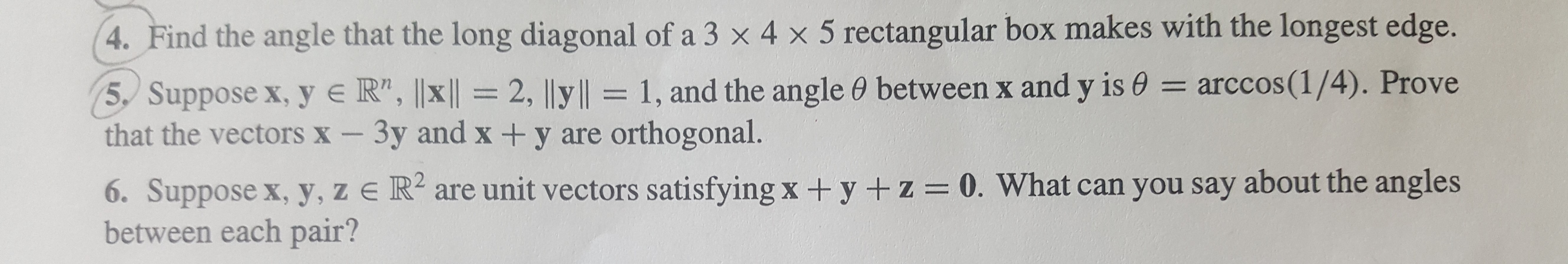4. Find the angle that the long diagonal of a 3 x 4 x 5 rectangular box makes with the longest edge.= arccos(1/4). Prove5, Suppose x, y e R", ||x ||= 2, |ly||= 1, and the angle 0 between x and y is ethat the vectors X - 3y and x + y are orthogonal.6. Suppose x, y, z e R are unit vectors satisfying x + y + z = 0. What can you say about the anglesbetween each pair?

Questionhelp_outlineImage Transcriptionclose4. Find the angle that the long diagonal of a 3 x 4 x 5 rectangular box makes with the longest edge. = arccos(1/4). Prove 5, Suppose x, y e R", ||x ||= 2, |ly||= 1, and the angle 0 between x and y is e that the vectors X - 3y and x + y are orthogonal. 6. Suppose x, y, z e R are unit vectors satisfying x + y + z = 0. What can you say about the angles between each pair? fullscreen
Step 1

We are given that the unit vectors x, y, z satisfies the equation

To find the relation between the relation between angles of each pair we have to take dot product with x, y, z

Step 2

By taking the dot products we get

While taking dot product consider the fact that all vectors are unity.

Step 3

Adding the three equations we get

From t...

Want to see the full answer?

See Solution

Want to see this answer and more?

Our solutions are written by experts, many with advanced degrees, and available 24/7

See Solution
Tagged in

Math